# Linear Resonant Response Regimes

Table 5.2: The various linear resonant response regimes. See Equations (4.65), (5.61), (5.69), (5.66), and (5.110)–(5.113). The response regimes are the resistive-inertial (RI), the viscous-resistive (VR), the semi-collisional (SC), the diffusive-resistive (DR), the inertial (I), the viscous-inertial (VI), and the diffusive-inertial (DI).
 Regime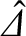Extent in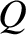-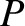space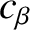limit RI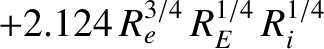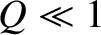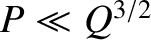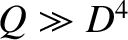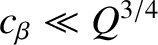VR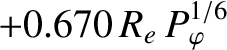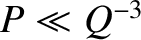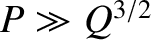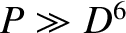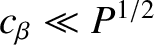SC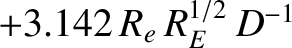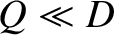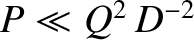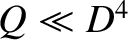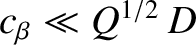DR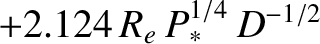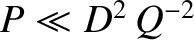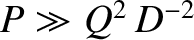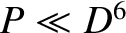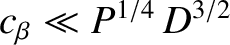I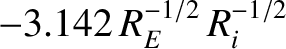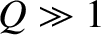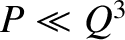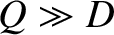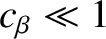VI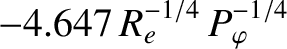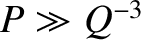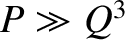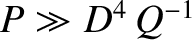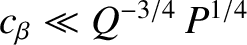DI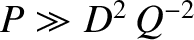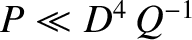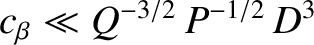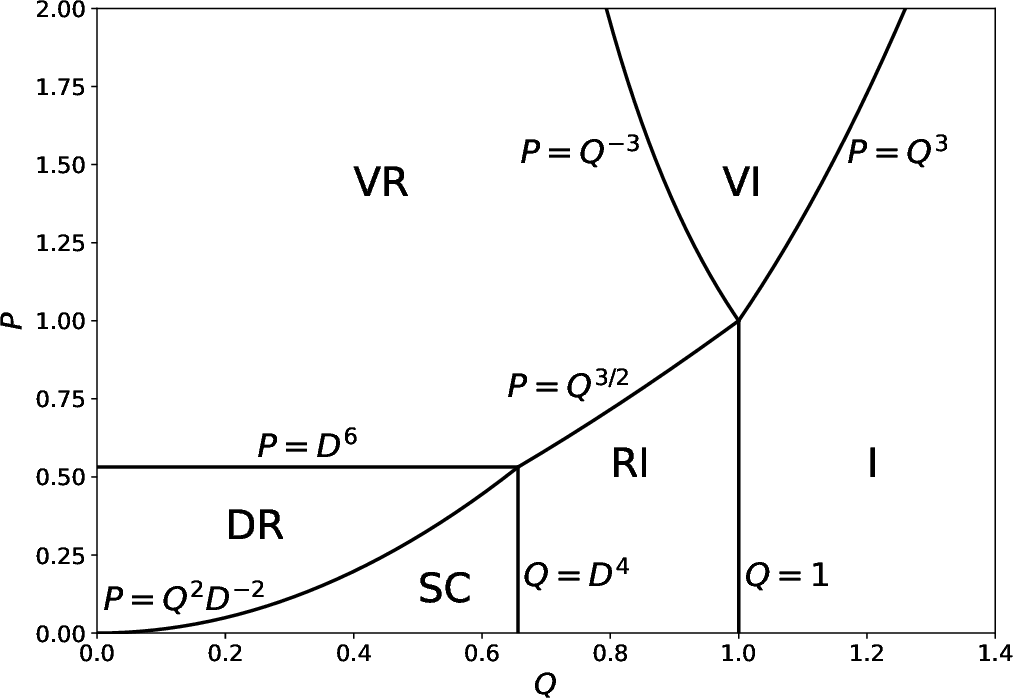Table 5.2 summarizes the properties of the various linear resonant response regimes found in Sections 5.9 and 5.11. Here, we have made use of the abbreviations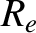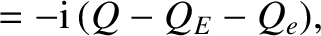(5.110)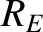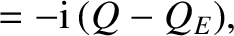(5.111)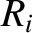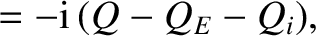(5.112)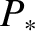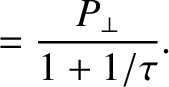(5.113)

In addition, Figures 5.1 and 5.2 show the extents of the various different response regimes in-space for the cases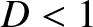and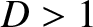, respectively.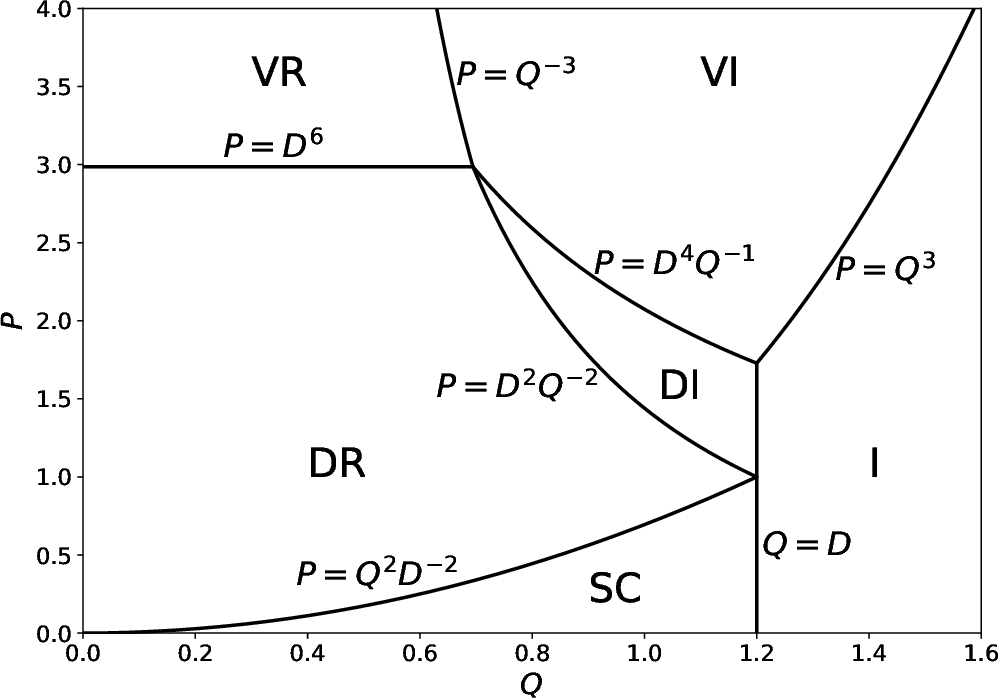Let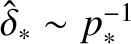be the normalized radial thickness of the resonant layer. Of course, the true thickness is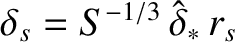. It follows from Equation (5.69) that the relative change in the perturbed helical magnetic flux,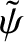, across the layer is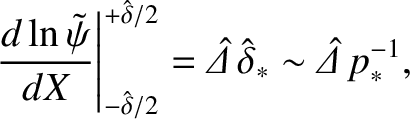(5.114)

According to the analysis of Section 5.9,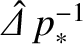takes the respective values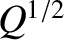,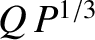,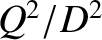, and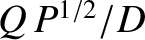in the resistive-inertial, viscous-resistive, semi-collisional, and diffusive-resistive response regimes. Moreover, it is clear from Figures 5.1 and 5.2 that these values are all much less than unity. In other words, it is indeed the case thatdoes not vary substantially across a “constant-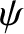” resonant layer. On the other hand, according to the analysis of Section 5.11,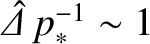in the inertial, viscous-inertial, and diffusive-inertial response regimes, which implies thatdoes vary substantially across a “nonconstant-” layer.